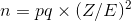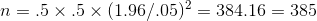# AP Statistics : Confidence and Proportion

## Example Questions

Explanation:

### Example Question #2 : How To Find Confidence Intervals For A Difference Between Two Proportions

In a simple random sample of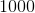people,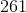are left-handed. Find a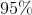confidence interval for the true proportion of left-handed people in the entire population.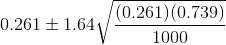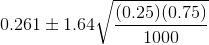The sample is not sufficiently large. A confidence interval cannot be used.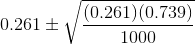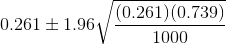Explanation:

Step 1: Determine the approporiate formula. In this case, the statistic is a proportion because the question says 261 people out of 1000 are left-handed. We want to use a confidence interval for proportions. The equation is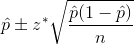Step 2: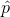, or the sample proportion is equal to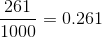Step 3: The questions asks for a 95% confidence interval. You can assume a normal distribution because of the large sample size. Therefore, in a normal distribution, 95% of the data is contained within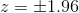.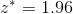Step 4: Substitue all the values into the equation to get the answer.### Example Question #1 : How To Find Confidence Intervals For A Proportion

A health insurance executive suspects that 50% of insurance applications are incomplete (i.e. critical data is missing from the application). He wants to gather a sample to estimate the true proportion. He wants to be within 5% of the true mean with 95% confidence.

What sample size does he need?

365

405

395

375

385